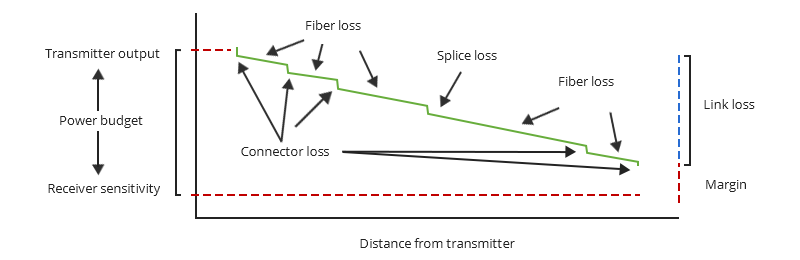Cancel

Change
English

### Explore

Change# Understanding Fiber Loss: What Is It and How to Calculate It?

April 17, 2020

##Accurate measurement and testing in fiber cable installation are crucial to ensure overall network integrity and performance. A significant signal loss in the optical fiber can cause unreliable transmission. How can we know the value of losses on the fiber link？Read on, this post will teach you how to calculate the losses in optical fiber and judge the fiber link performance.

## Types of Losses in Optical Fiber

What is optical fiber loss? Fiber loss can be also called fiber optic attenuation or attenuation loss, which measures the amount of light loss between input and output. Factors causing fiber loss are various, such as intrinsic material absorption, bending, connector loss, etc.

Losses in the optical fiber can be categorified into intrinsic optical fiber losses and extrinsic optical fiber loss depending on whether the loss is caused by intrinsic fiber characteristics or operating conditions. Intrinsic Optical Fiber Losses comprise of absorption loss, dispersion loss and scattering loss caused by the structural defects. Extrinsic Optical Fiber Losses contains splicing loss, connector loss, and bending loss. The detailed information about these optical losses and how to reduce them are introduced in How to Reduce Various Types of Losses in Optical Fiber?

## Standards for Fiber Loss

Telecommunications Industry Association (TIA)/Electronic Industries Alliance (EIA) develops TIA/EIA standards, which specify performance and transmission requirements for fiber optic cables, connectors, etc. and are widely accepted and used in the optical fiber industry. The maximum attenuation is actually the attenuation coefficient of fiber optic cable, which is expressed in dB/km units. It is one of the most important parameters for fiber loss measurement. According to TIA/EIA-568, the maximum attenuation for different types of fiber optic cables are shown in the following graph:

Cable Type Cable Type Wavelength (nm) Maximum Attenuation (dB/km) Minimum Transmission Capacity (Mhz * km）
50/125 micron multimode 850 3.5 500
1300 1.5 500
62.5/125 micron multimode 850 3.5 160
1300 1.5 500
Singlemode inside plant cable 1310 1.0 N/A
1550 1.0 N/A
Singlemode outside plant cable 1310 0.5 N/A
1550 0.5 N/A

## How to Calculate Losses in Optical Fiber?

To detect whether the link runs properly, the following calculation should be performed.

### Calculating Optical Fiber Losses

It is often the case to calculate the maximum signal loss across a given fiber link during optical cable installation. First, you should be aware of the fiber loss formula:

The Total Link Loss = Cable Attenuation + Connector Loss + Splice Loss

Cable Attenuation (dB) = Maximum Cable Attenuation Coefficient (dB/km) × Length (km)

Connector Loss (dB) = Number of Connector Pairs × Connector Loss Allowance (dB)

Splice Loss (dB) = Number of Splices × Splice Loss Allowance (dB)

As these formulas show, the total loss is the maximum sum of the worst variables within a fiber segment. It must be noted that the total loss calculated in this way is just an estimation that assumes the possible values of component losses so the actual loss could be higher or lower depending on various factors.

Let’s take a practical case as an example to demonstrate the calculation steps. There is a single mode fiber optic cable installed between two buildings, with a distance of 10km and the optical wavelength of 1310nm. The cable has 2 ST connector pairs and 1 splice.Figure 1: Calculate link loss over 10km SMF

• Calculate the fiber cable attenuation loss. According to the standard chart above, the light attenuation of 1310nm SM outside optical fiber cable is 0.5dB/km, therefore the total cable attenuation is 0.5dB/km × 10km = 5dB.

• Calculate the total connector loss. Use the TIA/EIA maximum loss per pair as 0.75 and the total connector loss is 0.75dB × 2 =1.5dB. In practical calculation, the actual connector loss can refer to the value in the fiber optic cable specifications provided by suppliers.

• Calculate the total splice loss. Use the TIA/EIA maximum loss as 0.3 per splice and the total splice loss is 0.3dB × 1=0.3dB.

• Calculate the other component loss if there are any other components such as attenuators.

• Add the cable loss, connector loss, and splice loss and get the total link loss. The total loss for this link is 5dB + 1.5dB + 0.3dB = 6.8dB.

Note that the estimates are just assumed, the easiest and most accurate way is to use OTDR trace of an actual link.

### Calculating Power Budget

How does this link loss value matter for the whole transmission? Here we have to mention another closely related term - power budget. It is used for comparison with the calculated total loss to ensure the cable plant is installed properly. The link will operate normally only when the link loss is within the loss budget. The power budget (PB) is calculated as the result of the difference between the sensitivity of the receiver (PR) and the output of the transmitter into the fiber (PT). The calculating formula is PB = PT - PR. Suppose the average transmitter output power is -15dBm, the receiver sensitivity is -28dBm, the power budget will be -15dB - (-28dB) = 13dB.

### Calculating Power Margin

After calculating the link loss and power budget, you can calculate the power margin, which is also referred to as safety margin. It represents the amount of power available after subtracting link loss from the power budget. The formula is PM = PB - LL.Figure 2: Power budget, link loss, and power margin

Take the 10km SMF case as an example, the power budget is 13dB and the link loss is known as 6.8dB, thus the safety budget is 13dB - 6.8dB = 6.2dB. The value greater than zero indicates that the link has sufficient power for transmission.

18.7k